Next: Geometrical analysis of imaging Up: 7: GEOMETRICAL PROBLEMS OF Previous: Interference analysis

## Untying loops

If, at direct propagating of a wave in a medium with velocity, one observes appearance of loops, then at reverse continuation of the wave into the same medium these loops will be untied. But usually the velocity functionis unknown. Moreover, we have to untie loops just in order to obtain good conditions for velocity estimation. So the question arises: how many continuation velocity vc can be different from real velocity without the loss of the untying effect?

I've obtained the following conditions:

1. The observed field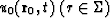is not complicated by loops if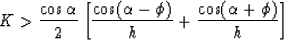holds for any ray, where K,h and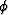are a curvature, a depth and a slope of the interface at the reflection point accordingly;is an angle of incident.

2. The condition for the absence of loops in the fieldcontinued (with respect to receivers) onto the horizontal plane z=hc with continued velocitybecomes weaker: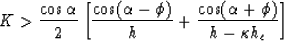(72)
where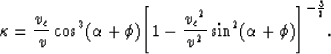3. In the case of double (with respect to receivers and sources) continuation we have the weakest condition:4. For small reflection angles the condition (72) may be simplified:where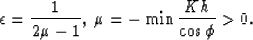This condition is shown in Figure. An example of untying loops is shown in Figurewhere data of physical modeling are represented.

We can interpret inverse wave-field continuation into the model with velocity v=vc as a continuation in a fictitious layer above the surface z=0 (the layer with known velocity).

The fact that at z>0 we have direct propagation and at z<0 reverse continuation causes the modification of Snell's law at the interface z=0. This modification is shown in Figure.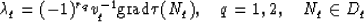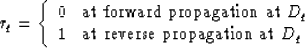Next: Geometrical analysis of imaging Up: 7: GEOMETRICAL PROBLEMS OF Previous: Interference analysis
Stanford Exploration Project
1/13/1998